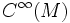# Algebra of infinitely differentiable functions

This article defines a basic construct that makes sense on any differential manifold
View a complete list of basic constructs on differential manifolds
This article gives a global construction for a differential manifold. There exists a sheaf analog of it, that associates a similar construct to every open subset. This sheaf analog is termed: sheaf of infinitely differentiable functions

## Definition

### Definition with symbols

Let$M$ be a differential manifold. The algebra of infinitely differentiable functions on$M$, denoted$C^\infty(M)$, is a commutative unital$\R$-algebra defined as follows:

• As a set, it is the set of all smooth maps from$M$ to$\R$. Equivalently, it is the set of those maps from$M$ to$\R$ whose restriction to any coordinate chart is smooth.
• The$\R$-algebra structure is by pointwise addition, multiplication and scalar multiplication.

A somewhat more useful gadget, particularly for comparing local and global information, is the sheaf of infinitely differentiable functions. This associates, to every open subset, the algebra of infinitely differentiable functions defined on that open subset.